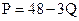Chapter 5, Problem 33P### Principles of Economics 2e

2nd Edition
Steven A. Greenlaw; David Shapiro
ISBN: 9781947172364

#### Solutions

Chapter
Section### Principles of Economics 2e

2nd Edition
Steven A. Greenlaw; David Shapiro
ISBN: 9781947172364
Textbook Problem

# The equation for a demand curve isWhat is the elasticity in moving from quantity of 5 to a quantity of 6?

To determine

Calculate the elasticity of demand for the following demand function when quantity rises from 5 units to 6 units.

P= 48 - 3Q.

Explanation

We will first find the price at quantity of 5 units.

P = 48 - 3QP = 48- 3×5P = 48 - 15P = $33 Now, we will find the price at quantity of 6 units. P = 48 - 3QP = 48- 3×6P = 48 - 18P =$30

Now, we can calculate the elasticity of demand with the foll

### Still sussing out bartleby?

Check out a sample textbook solution.

See a sample solution

#### The Solution to Your Study Problems

Bartleby provides explanations to thousands of textbook problems written by our experts, many with advanced degrees!

Get Started

#### Find more solutions based on key concepts